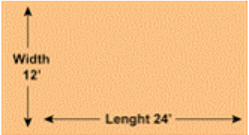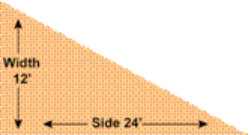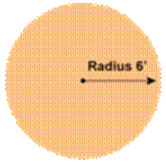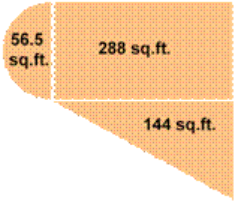# Resources

### What are the benefits of laying Sod?

Create your unique landscape plan by taking into consideration the things you love most. Sod works well with xeriscaping, rock, mulch, flower beds, trees, and fences. Plan your process so that you can access areas to be sodded easily, leave out fence panels where possible to allow forklift access for the sod. Forklifts will need about 10 ft access for placing pallets of sod in your yard.

### Installing a Sprinkler System

Classic Turf can recommend several professional sprinkler companies to help with this step. A well designed sprinkler system conserves water, and saves you time & money – also adding value to your home.
Here are some of the benefits of a sprinkler system: Good sprinkler systems apply water in a uniform, consistent and efficient manner. It allows you to water at optimal times: late in the evening, at night & early in the morning. When there is less evaporation and the water can soak in. It waters in multiple cycles to prevent any run-off, encouraging deep rooting for your Sod. Set the sprinkler to water when you are on vacation or away from home.

### Measuring For Sod

Measuring the amount of Sod you will need is really quite easy using the guidelines that we have provided below. These methods will give you the total amount needed in square feet. All of our sod is sold by the square foot (sq.ft.) so this will allow you to order just the right amount.

Step One:
On a piece of paper, draw a rough sketch of the areas to be sodded. Measure the areas and simply write down your measurements on the drawing. You can then break up the areas into the shapes below and figure out the square footage of each area. Then simply add up the measurements from the different shapes, and you will have the grand total of Sod that you will need.
*Note: Please remember that we can’t take back sod once it has left our farm or been delivered to you. In the event that you do run out, we always have more Sod. This guide will help you measure and order accurately. Please check your numbers twice. Classic Turf cannot take responsibility for footage figures unless we measure it ourselves. If you need help determining measurements on an unusually shaped or especially large project, let us know.

Square or Rectangle
This is the easiest shape to measure. Simply measure the Length & Width in square feet and multiply, as in the example below.
Length (24) x Width (12)
12 x 24 = 288 sq.ft. = 288 square feet is the Total Area to be SoddedRight Triangle Shape
A Right Triangle shape has one 90º corner. To determine the amount of Sod needed, multiply the two sides that contact the right angle and then divide by 2, as in the example below.
Length (24) x Width (12)
12 x 24 = 288 sq.ft. divided by 2 = 144 sq.ft. is the Total Area to be SoddedCircle Shape
To measure the area of a circle, first measure the radius of the circle (the radius is the distance from the center of the circle to the outside edge). Then multiply the radius times the radius, and then multiply that number by 3.14, as in the example below.
6 x 6 = 36 x 3.14 = 113 sq.ft. is the Total Area to be SoddedIrregular or Unusual Area Shapes
The measurement for irregular areas is simple to determine using a combination of the methods already described. Firstly, break the total space into smaller shapes, as illustrated below. Then determine the square footage of each of the smaller shapes. In the example below, the circle is cut in half, there’s a rectangle, and a triangle. Finally, add up all of the smaller areas for a grand total in sq.ft. (The sq.ft. for the circle is divided in 2 in the example below).
288 + 144 + 56.5 = 488.5 sq.ft. is the Total Area to be Sodded